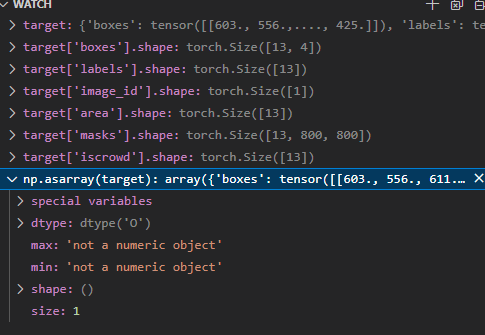# TypeError: can't convert np.ndarray of type numpy.object_. The only supported types are: float64, float32, float16, complex64, complex128, int64, int32, int16, int8, uint8, and bool

Hi, I am trying to create a segmentation model with a pretrained FCN ResNet50 model but I am getting an error from the segmentation reference code. I’m not sure if I am creating the targets correctly in my __getitem__ function in my dataloader class and was wondering if anyone could help.

I was trying to follow this tutorial PyTorch object detection model training from fiftyone. I know the tutorial is for detection but I thought I could adapt it to a segmentation model. I’m also using the semantic segmentation reference code in the main branch from the vision repo here. The error is happening in the transforms.py file from the reference in the PILToTensor class.

``````     78 def __call__(self, image, target):
79     image = F.pil_to_tensor(image)
---> 80     target = torch.as_tensor(np.array(target), dtype=torch.int64)
81     return image, target
``````

Here is my __getitem__ code for my custom Dataloader

``````    def __getitem__(self, idx):
img_path = self.img_paths[idx]
sample = self.samples[img_path]
print("frame_size", frame_size)
img = Image.open(img_path).convert("RGB")

boxes = []
labels = []
area = []
iscrowd = []
detections = sample[self.gt_field].detections
for det in detections:
category_id = self.labels_map_rev[det.label]
coco_obj = fouc.COCOObject.from_label(
)
x, y, w, h = coco_obj.bbox
boxes.append([x, y, x + w, y + h])
labels.append(coco_obj.category_id)
area.append(coco_obj.area)
iscrowd.append(coco_obj.iscrowd)

target = {}
target["boxes"] = torch.as_tensor(boxes, dtype=torch.float32)
target["labels"] = torch.as_tensor(labels, dtype=torch.int64)
target["image_id"] = torch.as_tensor([idx])
target["area"] = torch.as_tensor(area, dtype=torch.float32)
target["iscrowd"] = torch.as_tensor(iscrowd, dtype=torch.int64)